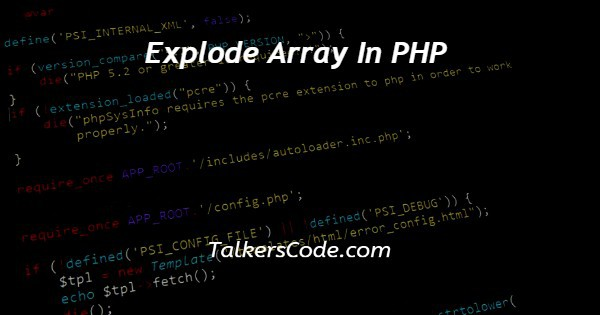# Explode Array In PHP

Last Updated : Sep 19, 2023In this article we will show you the solution of explode array in php, a built-in PHP method called explode() can be used to break a string into many strings. The explode() function divides a string depending on a string delimiter, i.e., whenever the delimiter character appears in the string.

An array of the strings produced by slicing the original string is the function's output.

Three parameters, two of which are required and one of which is optional, are accepted by the explode function.

When this character appears in a string, it denotes the beginning of one array element and the end of another, thus indicating the critical points or places at which the string will divide.

The input string that needs to be divided into an array is called OriginalString.

NoOfElements can be removed. It is employed to specify the array's element count.

Any integer, whether positive, negative, or zero, can represent this parameter. We'll talk about the PHP explode array concept now.

## Step By Step Guide On Explode Array In PHP :-

```<?php
\$NoOfElements = count(explode(" ",\$OriginalString));
echo "Exploding without optional parameter NoOfElements:";
print_r(explode(" ",\$OriginalString));
echo "Number of elements: " . \$NoOfElements . "\n\n";
\$NoOfElements = count(explode(" ",\$OriginalString,3));
echo "Exploding with positive NoOfElements:";
print_r(explode(" ",\$OriginalString,3));
echo "Number of elements: " . \$NoOfElements . "\n\n";
\$NoOfElements = count(explode(" ",\$OriginalString,-1));
echo "Exploding with negative NoOfElements:";
print_r(explode(" ",\$OriginalString,-1));
echo "Number of elements: " . \$NoOfElements . "\n\n";
?>```
1. You can see that we wrote PHP code to explode an array in this section. Utilising a chosen delimiter, the explode() method can divide a string into an array of substrings.
2. The variable \$OriginalString was given a string at the beginning of the code. The explode() function is then called three times with various inputs.
3. Whenever a space (which serves as the delimiter in this case) is encountered in a string, the first time explode() is called, it separates the string into an array of substrings, and the resulting array is displayed using the print_r() function.
4. The count() function is then used to determine how many elements there are in the resulting array, after which the number is written to the console.
5. Without utilising the optional argument \$NoOfElements, this is accomplished.
6. The text is divided into a maximum of three substrings when explode() is called a second time, and the resulting array is displayed to the terminal using print_r().
7. The count() function is used once again to count the entries in the resulting array, and the number is then displayed to the console.
8. The optional \$NoOfElements parameter, which is set to 3, is used to do this.
9. The string is divided into as many substrings as possible the third time explode() is used, with the exception of the final substring, which is omitted from the resultant array.
10. Using print_r(), the resultant array is printed to the console along with its element count, which is calculated using count() and also printed to the console.
11. The optional \$NoOfElements parameter, which is set to -1, is used to do this.
12. Before each call to the print_r() function in the final line, we use the echo statement to print a message to the console that identifies the call to explode() that is being made.
13. The size of the generated array is also included in this message.

## Conclusion :-

As a result, we were able to understand the PHP explode array notion.

Additionally, we discovered how helpful the PHP explode() function is for breaking up strings into an array of substrings based on a given delimiter.

This piece of code shows how to modify the behavior of the function explode() by passing it various parameters.

The user can select the most number of elements to return in the final array by using the optional parameter \$NoOfElements.

I hope this article on explode array in php helps you and the steps and method mentioned above are easy to follow and implement.